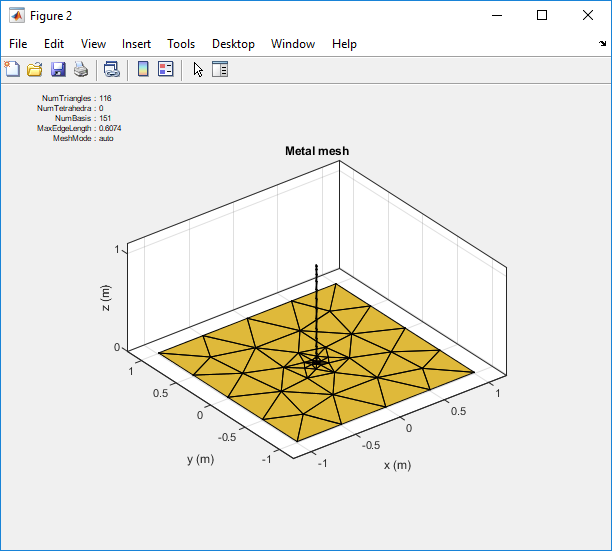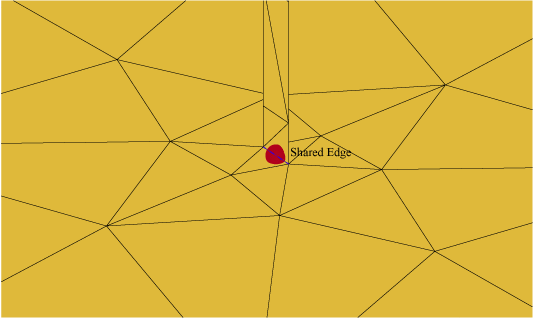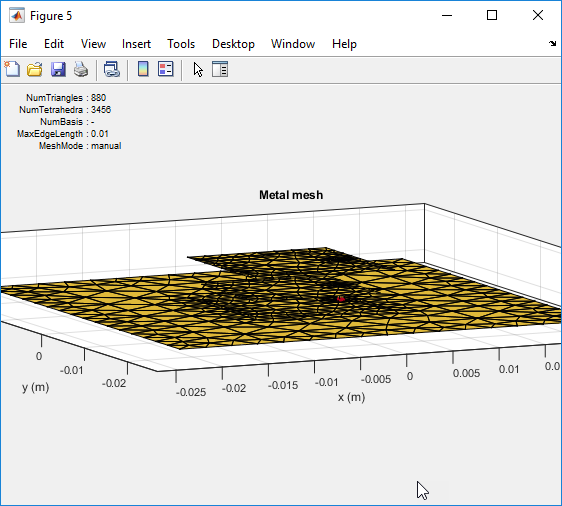## Feed Model

An antenna feed usually uses a conventional transmission line through two electrically close terminals. Feed model and fed location are very important variables in antenna analysis as the input impedance at the feed location affects the resulting radiation pattern directivity and gain of the antenna. An antenna can be fed using a open-wire transmission line or a coaxial feed through ground plane. These feed systems directly impact the antenna impedance characteristics. Antenna in Antenna Toolbox™ uses the delta-gap source feed model.

### Delta-Gap Source Feed Model

Delta-gap source models the feed assuming that the electric field exists only in the small gap between the terminals. In the case of RWG edge elements, the gap field is described using a delta-function generator or feeding edge model. This model assumes that the excitation voltage at the feed terminals is of a constant value Vi and zero else. The incident electric field is defined as:

`$E=-\nabla \phi =\frac{V}{\Delta }\text{n}{}_{y}$`

where:

• φ - Electric potential

• V - Voltage across the gap

• Δ - Gap width

. This electric field is also constant at the feed terminals and zero elsewhere.

### Single-Edge Delta Source Feed Models

#### Dipole Antenna

Create a dipole antenna using the `dipole` object and mesh the antenna.

```ant = dipole; i = impedance(ant,75e6) mesh(ant)```Zoom in on the feed region of the antenna. You will see that feed point shares one common edge. In the case of the dipole, this is where the gap exists. Here the voltage is 1 and the phase applied is 0 degrees. Everywhere else on the dipole the voltage will be zero.#### Monopole Antenna

Create a monopole antenna using the `monopole` object and mesh the antenna.

```ant = monopole; i = impedance(ant,75e6) mesh(ant)```Zoom in on the feed region of the antenna. You will see that feed point shares one common edge between the vertical radiator and the groundplane. In the case of the monopole, this is where the gap exists. Here the voltage is 1 and the phase applied is 0 degrees. Everywhere else on the dipole the voltage will be zero.### Multi-Edge Delta Source Feed Models

You can also improve on the single edge feed by creating more accurate multi-edge feeds using `pcbStack`.Consider the above mesh for a stacked patch antenna with solid-square feed model. Zoom in on the feed model. You see that the solid-square feed have four edges connected to the ground plane. This feed-model increases the number of unknowns but attempts to more accurately represent the feed geometry.

Note

To create the stacked patch antenna, see Modeling and Analysis of a Probe-Fed Stacked Patch Antenna.